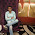## Dec 10, 2009

### Infinity Problem

Suppose you have a hotel which has one floor with infinite number of rooms in a row and all of them are occupied. A new customer wants to check in, how will you accommodate her? What if infinite number of people want to check in, how will you accommodate them then?

Hint: Define infinity. :)

Update (18/12/09): Solution in comments by Nikhil Pandey (CSE, IITB alumnus)

1.1) ask everyone to move to next room
2) ask everyone to move from x->2x

????

2.pandeyji.. (@Gold and Iron)

Since there are infinite number of rooms and infinite+1= infinite
Just ask person in room k to move to k+1. Thus making a room in the first room. :)

In the other case, since infinite+infinite = infinite
asking person in room k to move to 2k solves the problem.

3.Third part: Suppose infinite number of buses arrive at the hotel, each having infinite number of people, how will you accommodate them? :)

4.@Sherminator..
Nice one..
Since NxN is countable set. We can get a one to one mapping from N to NxN
Hence, we can accommodate (infinite people X infinite buses) in the hotel.

Relevant article:

http://en.wikipedia.org/wiki/Cantor_pairing_function

Thanks!

5.@Pratik . Infinite number of buses having infinite number of people would make the set N^N i.e. N x N x N..... N times...

6.Infinite Cartesian product of countable sets is uncountable

7.Oh got it . Its a countable union of countable set.

### Fraction Brainteaser

Source: Sent to me by Gaurav Sinha Problem: Siddhant writes a Maths test and correctly answers 5 out of 6 Arithmetic questions and 20...